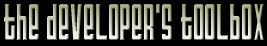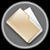This section of the archives stores flipcode's complete Developer Toolbox collection, featuring a variety of mini-articles and source code contributions from our readers.Octree Implementation   Submitted byEditor's Note: This code compliments an Ask MidNight response on octrees which can be found here.

 ``` // COTD Entry submitted by Paul Nettle [midnight@FluidStudios.com] // Corresponds with an Ask MidNight response (http://www.flipcode.com/askmid/) // ----------------------------------------------------------------------------- // This defines a callback for traversal // ----------------------------------------------------------------------------- class Octree; typedef bool (*callback)(const Octree &o, void *data);// ----------------------------------------------------------------------------- // This defines a point class (it's incomplete, but the data elements are there) // ----------------------------------------------------------------------------- class Point { public: double x, y, z; // Position double n; // User's unique identifier unsigned int code; // Used during octree generation // Insert typical operators, such as *, +, -, etc. };// ----------------------------------------------------------------------------- // This defines a cubic bounding volume (center, radius) // ----------------------------------------------------------------------------- typedef struct { Point center; // Center of a cubic bounding volume double radius; // Radius of a cubic bounding volume } Bounds;// ----------------------------------------------------------------------------- // The octree class -- almost real code! // ----------------------------------------------------------------------------- class Octree { public: // Construction/Destruction Octree(); virtual ~Octree(); // Accessors inline const Point * const * points() const {return _points;} inline const unsigned int pointCount() const {return _pointCount;} // Implementation virtual const bool build(Point **points, const unsigned int count, const unsigned int threshold, const unsigned int maximumDepth, const Bounds &bounds, const unsigned int currentDepth = 0); static const Bounds calcCubicBounds(const Point * const * points, const unsigned int count); virtual const bool traverse(callback proc, void *data) const;protected: Octree *_child; unsigned int _pointCount; Point **_points; Point _center; double _radius; };// ----------------------------------------------------------------------------- // Construction -- Just "nullify" the class // ----------------------------------------------------------------------------- Octree::Octree() : _pointCount(0), _points(NULL), _center(0,0,0,0), _radius(0.0) { memset(_child, 0, sizeof(_child)); }// ----------------------------------------------------------------------------- // Destruction -- free up memory // ----------------------------------------------------------------------------- Octree::~Octree() { delete[] _points; }// ----------------------------------------------------------------------------- // Build the octree // ----------------------------------------------------------------------------- const bool Octree::build(Point **points, const unsigned int count, const unsigned int threshold, const unsigned int maximumDepth, const Bounds &bounds, const unsigned int currentDepth) { // You know you're a leaf when... // // 1. The number of points is <= the threshold // 2. We've recursed too deep into the tree // (currentDepth >= maximumDepth) // // NOTE: We specifically use ">=" for the depth comparison so that we // can set the maximumDepth depth to 0 if we want a tree with // no depth. if (count <= threshold || currentDepth >= maximumDepth) { // Just store the points in the node, making it a leaf _pointCount = count; _points = new Point *[count]; memcpy(_points, points, sizeof(Point *) * count); return true; } // We'll need this (see comment further down in this source) unsigned int childPointCounts; // Classify each point to a child node for (unsigned int i = 0; i < count; i++) { // Current point Point &p = *points[i]; // Center of this node const Point &c = bounds.center; // Here, we need to know which child each point belongs to. To // do this, we build an index into the _child[] array using the // relative position of the point to the center of the current // node p.code = 0; if (p.x > c.x) p.code |= 1; if (p.y > c.y) p.code |= 2; if (p.z > c.z) p.code |= 4; // We'll need to keep track of how many points get stuck in each // child so we'll just keep track of it here, since we have the // information handy. childPointCounts[p.code]++; } // Recursively call build() for each of the 8 children for (i = 0; i < 8; i++) { // Don't bother going any further if there aren't any points for // this child if (!childPointCounts[i]) continue; // Allocate the child _child[i] = new Octree; // Allocate a list of points that were coded JUST for this child // only Point **newList = new Point *[childPointCounts[i]]; // Go through the input list of points and copy over the points // that were coded for this child Point **ptr = newList; for (unsigned int j = 0; j < count; j++) { if (points[j]->code == i) { *ptr = points[j]; ptr++; } } // At this point, we have a list of points that will belong to // this child node. We'll want to remove any point with a // duplicate 'n' in them... // // [We won't need to reallocate the list, since it's temporary] int newCount = 0; for (j = 0; j < childPointCounts[i]; j++) { // Remove duplicates from newList // ... // Keep track of the new count in 'newCount' } // Generate a new bounding volume -- We do this with a touch of // trickery... // // We use a table of offsets. These offsets determine where a // node is, relative to it's parent. So, for example, if want to // generate the bottom-left-rear (-x, -y, -z) child for a node, // we use (-1, -1, -1). // // However, since the radius of a child is always half of its // parent's, we use a table of 0.5, rather than 1.0. // // These values are stored the following table. Note that this // won't compile because it assumes Points are structs, but you // get the idea. Point boundsOffsetTable = { {-0.5, -0.5, -0.5}, {+0.5, -0.5, -0.5}, {-0.5, +0.5, -0.5}, {+0.5, +0.5, -0.5}, {-0.5, -0.5, +0.5}, {+0.5, -0.5, +0.5}, {-0.5, +0.5, +0.5}, {+0.5, +0.5, +0.5} }; // Calculate our offset from the center of the parent's node to // the center of the child's node Point offset = boundsOffsetTable[i] * bounds.radius; // Create a new Bounds, with the center offset and half the // radius Bounds newBounds; newBounds.radius = bounds.radius * 0.5; newBounds.center = bounds.center + offset; // Recurse _child[i]->build(newList, newCount, threshold, maximumDepth, newBounds, currentDepth+1); // Clean up delete[] newList; } return true; }// ----------------------------------------------------------------------------- // Determine the [cubic] bounding volume for a set of points // ----------------------------------------------------------------------------- const Bounds Octree::calcCubicBounds(const Point * const * points, const unsigned int count) { // What we'll give to the caller Bounds b; // Determine min/max of the given set of points Point min = *points; Point max = *points; for (unsigned int i = 1; i < count; i++) { const Point &p = *points[i]; if (p.x < min.x) min.x = p.x; if (p.y < min.y) min.y = p.y; if (p.z < min.z) min.z = p.z; if (p.x > max.x) max.x = p.x; if (p.y > max.y) max.y = p.y; if (p.z > max.z) max.z = p.z; } // The radius of the volume (dimensions in each direction) Point radius = max - min; // Find the center of this space b.center = min + radius * 0.5; // We want a CUBIC space. By this, I mean we want a bounding cube, not // just a bounding box. We already have the center, we just need a // radius that contains the entire volume. To do this, we find the // maxumum value of the radius' X/Y/Z components and use that b.radius = radius.x; if (b.radius < radius.y) b.radius = radius.y; if (b.radius < radius.z) b.radius = radius.z; // Done return b; }// ----------------------------------------------------------------------------- // Generic tree traversal // ----------------------------------------------------------------------------- const bool Octree::traverse(callback proc, void *data) const { // Call the callback for this node (if the callback returns false, then // stop traversing. if (!proc(*this, data)) return false; // If I'm a node, recursively traverse my children if (!_pointCount) { for (unsigned int i = 0; i < 8; i++) { // We store incomplete trees (i.e. we're not guaranteed // that a node has all 8 children) if (!_child[i]) continue; if (!_child[i]->traverse(proc, data)) return false; } } return true; } ```